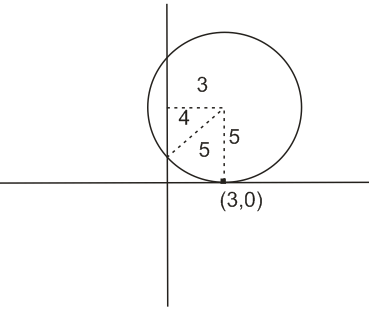# A circle touching the x-axis at (3, 0) and making an intercept of length 8 on the y-axis passes through the point: Option 1) (3,10) Option 2) (3,5)   Option 3) (2,3)   Option 4) (1,5)=> equation of circle is

Hence (3,10) will satisfy the equation.

Option 1)

(3,10)

Option 2)

(3,5)

Option 3)

(2,3)

Option 4)

(1,5)

Exams
Articles
Questions